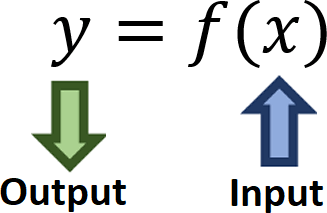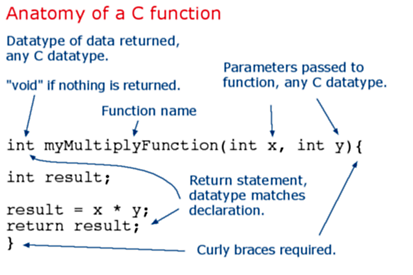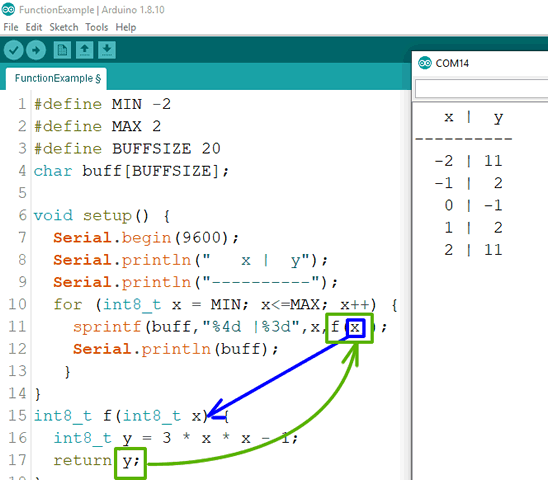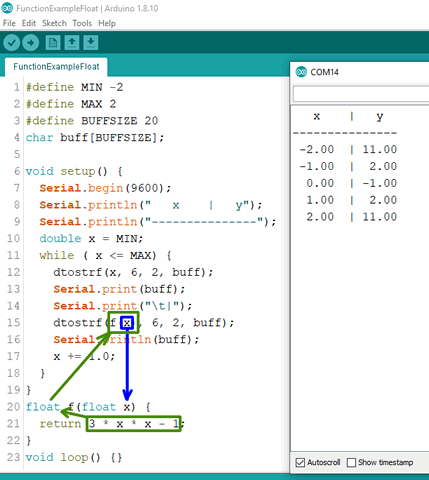Introduction to Functions in Arduino C

Web: Arduino: Functions
Reference: AVR Foundations. p. 45.Many of the subtasks your code is required to perform is best developed in short, modular chunks, called functions (aka methods, subroutines). In this way, it is both easy to identify and repair errors as well as reuse the code in future projects.

Your mathematical understanding of a function, that returns a single value within the range of the function is a good place to start. The notation to the right is understood to mean that a value x, chosen from the eligible domain, is the input to the function. The expression defined by f replaces x with this input value and the expression is evaluated and returned to you as an element of the range, y.

The familiar setup() and loop() function headers are preceded by the void keyword because they are intended to perform a task, not return a single value. If the purpose of a function is to perform a calculation on one or more input values, the function header would replace the void keyword with another valid data type and use the return statement to both exit the function and pass back the calculated result.Functions in Arduino C, and (almost) any other other language, work in a similar manner. A call to a function can appear almost anywhere you would include a constant or a variable.

Example 1. Let's say in order to graph the function f(x)=3x^2-1 in your notebook, you decide first create a table of values over the limited integer domain, [2,2]. You could easily do this by hand, but you want to try your hand at writing code to do it for you. You could write this.1. The use of sprintf is optional, but preferred for formatted output usage.
2. It was not explicitly necessary in this context to declare a local variable y in line 16 and then simply return it in the next statement (although it supports clarity) This evaluation and exit could have been done in one return statement.
3. This example must allow for the use of negative numbers so we choose int8_t over uint8_t for both domain and range values. However...
4. ...Since we know the domain of this quadratic function is all real numbers we should not have used integer types at all. We should have use Arduino's float type to allow for decimal places.

Example 2. Consider this improved version of Example 1...1. for loops are simply consolidated while loops (initialization, terminal, and step in one line) and are encouraged for the iteration of ordinal sequences of integer or character types.
2. However, for floating point iteration (float or double types) the formal while syntax is preferred.
3. Note in Lines 10 and 11 the compiler had no problem 'promoting' the integer variables MIN and MAX to float (it appended .0).
4. The developers of Arduino C provide limited support for the float type due to the heavy demands it places on Flash memory. Consequently, I've shown you an alternative way to provide formatted support for float types using the dtostrf() function. Overkill perhaps, but this at least gives you control over the number of desired decimal places.
5. Line 20 replaces the integer input parameter type and return type with float (7 decimal places of accuracy). Within the Arduino C world, the double type is the same as float.
6. Line 21 shows the elimination of the intermediate variable, y. The expression is evaluated and returned in a single statement.

Exercises

Create an Arduino project called Functions, and add functions to realize each of the following requirements. Be sure to consider the best return type and thoroughly test each function by displayed results to the Serial Monitor.

1. Develop a function maximum, that accepts two uint8_t parameters and returns the larger of the two.
2. Add a new maximum function that returns the larger of three uint8_t input parameters.
3. Develop a function product that accepts two int8_t parameters and returns the product of the two.
4. Develop a function disc that accepts the a, b and c values of a quadratic expression ax^2+bx+c and returns the value of the discriminant, b^2-4ac.
5. Develop the function dec2BCD that accepts a decimal value from 0 to 99, inclusive and returns its BCD equivalent.
6. A predicate method returns a boolean result. Develop the predicate method isEven that returns true if the uint16_t input parameter is even and false otherwise.
7. More to come...